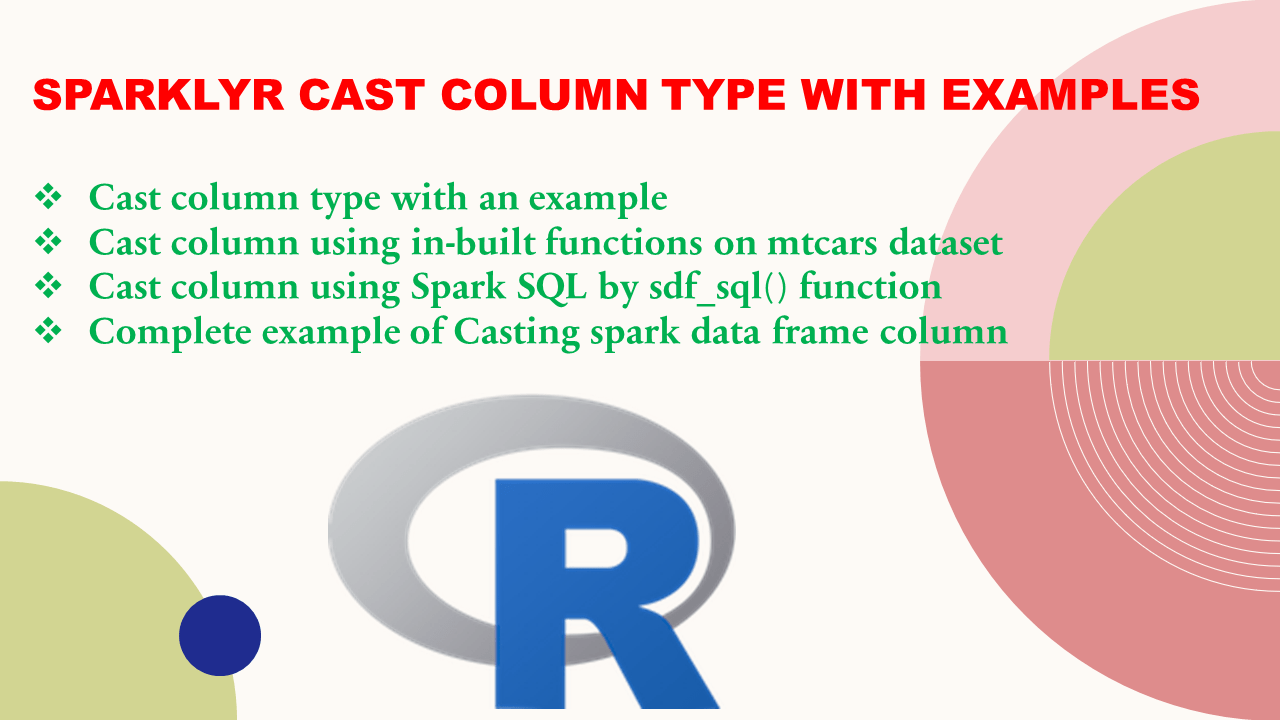# Sparklyr Cast Column Type With Examples

To convert or cast column type in Sparklyr use `as.double(), as.integer(), as.logical(), as.character(), as.date()`.

Sparklyr is an R package developed by RStudio that lets you analyze the data in Apache Spark while using other well-known R packages. In this article, we will see how you can use sparklyr to cast the datatype of data frame columns.

In sparklyr, there are in-built functions available to cast the data type of data frame columns from one data type to another. Moreover, you can use the `sdf_sql()` function to run a custom spark SQL expression to convert the dataset.

## 1. Cast column type with an example

Below are some examples that convert the Numeric/Double type to Integer and the Numeric/Double type to String/Character. %>% is a pipe operator in R that is from magrittr which takes the result of a first function as an argument to the next function/operation.

``````
# convert Integer to string/character
df %>% mutate(cyl = as.character(cyl))

#convert chracter to Integer and numeric
df %>% mutate(cyl = as.integer(cyl))
df %>% mutate(cyl = as.numeric(cyl))

# Using sdf_sql
sdf_sql("select CAST(cyl AS STRING) AS cyl, mpg, drat from mtcars")

``````

Below is the sample dataset available in-built in R with the datasets library.

`datasets` is an in-built package that comes with R programming which provides a total of 104 built-in datasets to work with. This is a very handy package when you want to practice with different datasets without worrying about downloading. In this article, we will use mtcars dataset to cast various columns using sparklyr.

``````
library(datasets)
mtcars_df <- mtcars

# To see the first 5 rows of the dataset
mtcars_df %>%
View()
``````

Output:

## 2. Cast column using in-built functions on mtcars dataset

Use `as.character()` function to convert the data type from any other data type to a character/string

Use `as.numeric() `function to convert the data type from any other data type to double

Use `as.integer()` function to convert the data type from any other data type to an integer

Use `as.logical()` function to convert the data type from any other data type to boolean

Use `as.date()` function to convert the data type from any other data type to date

Below sparklyr code snippet below casts the data frame column, cyl to string (character), gear to integer, and then again converts back to numeric.

``````
library(sparklyr)
library(dplyr)

# initiate spark connection
sc <- spark_connect(master = 'local',
spark_home = Sys.getenv("SPARK_HOME"),
app_name = "SparkByExamples.com",
method = 'shell',
version = '3.0.0')

#copy r local data frame to spark memory
mtcars_spark_df <- copy_to(sc, mtcars_df , "mtcars")

# using in-built functions
mtcars_r_df %
mutate(cyl = as.character(cyl)) %>%
mutate(gear = as.integer(gear)) %>%
mutate(gear = as.numeric(gear)) %>%
collect()

# view the structure of the dataframe with datatype of each column
str(mtcars_r_df)
``````

Output:

## 3. Cast column using Spark SQL by sdf_sql() function

`sdf_sql()` is a function available in sparklyr that we can use to cast spark DataFrame column “cyl” from Integer to String, “gear” from numeric to an integer to numeric again.

``````
# run custom spark SQL to cast multiple data frame columns
mtcars_df <- sdf_sql("select CAST(cyl AS STRING) AS cyl, mpg, drat from mtcars")
mtcars_r_df  <- mtcars_df %
dplyr::collect()

# View the structure of the data frame after casting columns
str(mtcars_r_df)
``````

## 4. Complete example of Casting spark data frame column

``````
library(sparklyr)
library(dplyr)
mtcars_df %
View()

# initiate spark connection using spark_connect
sc <- spark_connect(master = 'local',
spark_home = Sys.getenv("SPARK_HOME"),
app_name = "SparkByExamples.com",
method = 'shell',
version = '3.0.0')
mtcars_spark_df <- copy_to(sc, mtcars_df , "mtcars")
# using in-built functions
mtcars_r_df %
mutate(cyl = as.character(cyl)) %>%
mutate(gear = as.integer(gear)) %>%
mutate(gear = as.numeric(gear)) %>%
collect()

# View the structure of the data frame after casting columns
str(mtcars_r_df)

# using spark SQL
mtcars_df <- sdf_sql("select CAST(cyl AS STRING) AS cyl, mpg, drat from mtcars")

# View the data frame in new tab
View(mtcars_r_df)

# View the structure of the data frame after casting columns
str(mtcars_r_df)
``````

## Conclusion

In this article, you have learned how to cast the data types of sparklyr data frame column from one data type to another using in-built functions `as.integer(), as.numeric(), as.character()` and Spark SQL using `sdf_sql()`.

Happy sparklyr Learning!!

## Reference

https://spark.rstudio.com/